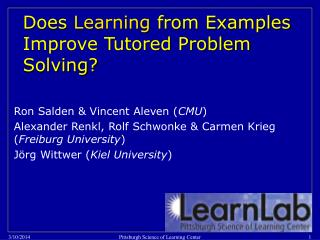# Does Learning from Examples Improve Tutored Problem Solving? - PowerPoint PPT PresentationDownload PresentationDoes Learning from Examples Improve Tutored Problem Solving?

Does Learning from Examples Improve Tutored Problem Solving?
Download Presentation## Does Learning from Examples Improve Tutored Problem Solving?

- - - - - - - - - - - - - - - - - - - - - - - - - - - E N D - - - - - - - - - - - - - - - - - - - - - - - - - - -
##### Presentation Transcript

1. Does Learning from Examples Improve Tutored Problem Solving? Ron Salden & Vincent Aleven (CMU) Alexander Renkl, Rolf Schwonke & Carmen Krieg (Freiburg University) Jörg Wittwer (Kiel University) Pittsburgh Science of Learning Center

2. Theory Combination of two successful research traditions • Cognitive Tutors • Worked Out Examples Pittsburgh Science of Learning Center

3. Scientific and applied questions • Scientific question Under what conditions can Tutored learning from examples be as effective or more effective than standard Tutored learning? • Applied question Can we speed up learning while retaining the learning outcomes? Pittsburgh Science of Learning Center

4. Placing Study within Robust Learning Framework • Primary research cluster • Interactive communication, coordinative learning, refinement & fluency • Independent variable(s) • IC: Reflective dialog, scripting collaboration, peer tutoring, peer observation of tutoring, varying contribution of student and system… • CL: self-explanation, integrate conceptual & procedural, fading of worked out steps, multi-modal… • R&F: Feature focusing, example comparison, cognitive mastery, optimal spacing, … • Dependent variables used • Normal post-test, long term retention, transfer measuring procedural and conceptual knowledge, future learning Pittsburgh Science of Learning Center

5. Control: standard problem solving with self explanation Contiguous interface:student works in diagram, entries appear in table after submitting answers and rules to the diagram Clicking on the question mark opens a work field Pittsburgh Science of Learning Center

6. Givens in problem statement are completely worked out to avoid extraneous load Formula and answer are worked out but student still has to find out what rule is being applied on this step Example condition: Worked out answer steps Pittsburgh Science of Learning Center

7. The sequencing of problems and fading of worked-out steps Note. W stands for worked out examples and S for problem solving Pittsburgh Science of Learning Center

8. Dependent variables Transfer test measuring: • procedural knowledge - problem solving and reasoning • conceptual knowledge - drawing problems - Multiple choice questions concerning geometric principles Note: hard to do long term retention test in lab study, we will include this in upcoming in vivo studies Pittsburgh Science of Learning Center

9. Main results lab study 1 • Example condition spent less time (t(48)= -3.11, p < .001, r = .41) to obtain the same learning outcomes as the control condition • Using time on Tutor: Higher learning efficiency for the example condition on transfer test measuring conceptual knowledge (t(48)= 1.73, p < .05, r = .24) and procedural knowledge (t(48)= 1.82, p < .05, r = .25) Pittsburgh Science of Learning Center

10. Main results lab study 2  Possible confounder Study 1: German participants inexperienced with Cognitive Tutor, thus more detailed instructions were given in Study 2 • Example condition showed higher gain on transfer test for conceptual understanding (sense-making) than control condition (t(28)= 1.85, p < .05, r = .33) Pittsburgh Science of Learning Center

11. Main results lab study 2 continued • Example condition spent less time (t(28)= -3.14, p < .001, r = .51) to complete the curriculum than control condition • Using time on Tutor: Higher learning efficiency for example condition on transfer test measuring conceptual knowledge (t(28)= 3.48, p < .001, r = .55) Pittsburgh Science of Learning Center

12. Interpretation of Results within Robust Learning Framework • Worked out examples lead to same level of foundational skillsin less time • Furthermore, study 2 shows that fading worked out examples can improve sense-making and consequently lead to better robust learning Pittsburgh Science of Learning Center

13. Reported lab studies, other LearnLab studies by McLaren et al, Ringenberg et al. Anderson et al. Sweller et al., Renkl & Atkinson Ongoing + upcoming studies • Results LearnLab studies with Tutor different than results in literature with no Tutor • Possibly the standard Cognitive Tutor is “too supportive” which cancels out potential effects of Example enriched Tutor • Conducting lab experiment with Cognitive Tutor resembling problem solving format used in literature (both Tutor conditions completed November 30) Pittsburgh Science of Learning Center

14. LearnLab experiment with adaptive fading of worked out steps -fading occurs based on student’s explanations -delayed post test incl. not enough info items to test long term retention Start date: January 11, 2007 Upcoming studies continued Pittsburgh Science of Learning Center